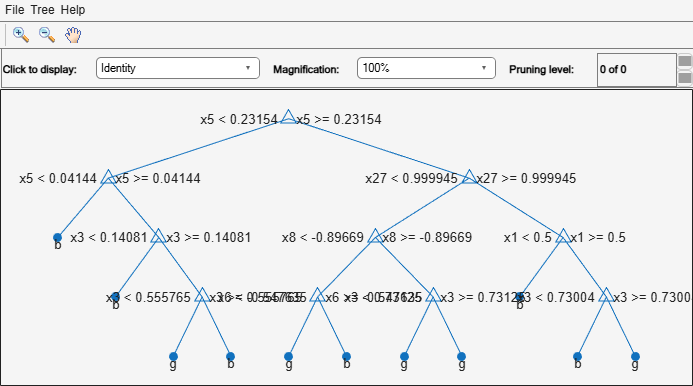Documentation

# CompactClassificationEnsemble

Package: classreg.learning.classif

Compact classification ensemble class

## Description

Compact version of a classification ensemble (of class `ClassificationEnsemble`). The compact version does not include the data for training the classification ensemble. Therefore, you cannot perform some tasks with a compact classification ensemble, such as cross validation. Use a compact classification ensemble for making predictions (classifications) of new data.

## Construction

```ens = compact(fullEns)``` constructs a compact decision ensemble from a full decision ensemble.

### Input Arguments

 `fullEns` A classification ensemble created by `fitcensemble`.

## Properties

 `CategoricalPredictors` Categorical predictor indices, specified as a vector of positive integers. `CategoricalPredictors` contains index values corresponding to the columns of the predictor data that contain categorical predictors. If none of the predictors are categorical, then this property is empty (`[]`). `ClassNames` List of the elements in `Y` with duplicates removed. `ClassNames` can be a numeric vector, vector of categorical variables, logical vector, character array, or cell array of character vectors. `ClassNames` has the same data type as the data in the argument `Y`. (The software treats string arrays as cell arrays of character vectors.) `CombineWeights` Character vector describing how `ens` combines weak learner weights, either `'WeightedSum'` or `'WeightedAverage'`. `Cost` Square matrix, where `Cost(i,j)` is the cost of classifying a point into class `j` if its true class is `i` (the rows correspond to the true class and the columns correspond to the predicted class). The order of the rows and columns of `Cost` corresponds to the order of the classes in `ClassNames`. The number of rows and columns in `Cost` is the number of unique classes in the response. This property is read-only. `ExpandedPredictorNames` Expanded predictor names, stored as a cell array of character vectors. If the model uses encoding for categorical variables, then `ExpandedPredictorNames` includes the names that describe the expanded variables. Otherwise, `ExpandedPredictorNames` is the same as `PredictorNames`. `NumTrained` Number of trained weak learners in `ens`, a scalar. `PredictorNames ` A cell array of names for the predictor variables, in the order in which they appear in `X`. `Prior` Numeric vector of prior probabilities for each class. The order of the elements of `Prior` corresponds to the order of the classes in `ClassNames`. The number of elements of `Prior` is the number of unique classes in the response. This property is read-only. `ResponseName` Character vector with the name of the response variable `Y`. `ScoreTransform` Function handle for transforming scores, or character vector representing a built-in transformation function. `'none'` means no transformation; equivalently, `'none'` means `@(x)x`. For a list of built-in transformation functions and the syntax of custom transformation functions, see `fitctree`. Add or change a `ScoreTransform` function using dot notation: `ens.ScoreTransform = 'function'` or `ens.ScoreTransform = @function` `Trained` A cell vector of trained classification models. If `Method` is `'LogitBoost'` or `'GentleBoost'`, then `CompactClassificationEnsemble` stores trained learner `j` in the `CompactRegressionLearner` property of the object stored in `Trained{j}`. That is, to access trained learner `j`, use `ens.Trained{j}.CompactRegressionLearner`.Otherwise, cells of the cell vector contain the corresponding, compact classification models. `TrainedWeights` Numeric vector of trained weights for the weak learners in `ens`. `TrainedWeights` has `T` elements, where `T` is the number of weak learners in `learners`. `UsePredForLearner` Logical matrix of size `P`-by-`NumTrained`, where `P` is the number of predictors (columns) in the training data `X`. `UsePredForLearner(i,j)` is `true` when learner `j` uses predictor `i`, and is `false` otherwise. For each learner, the predictors have the same order as the columns in the training data `X`. If the ensemble is not of type `Subspace`, all entries in `UsePredForLearner` are `true`.

## Methods

 edge Classification edge loss Classification error margin Classification margins predict Classify observations using ensemble of classification models predictorImportance Estimates of predictor importance for classification ensemble of decision trees removeLearners Remove members of compact classification ensemble

## Copy Semantics

Value. To learn how value classes affect copy operations, see Copying Objects (MATLAB).

## Examples

collapse all

Create a compact classification ensemble for efficiently making predictions on new data.

Load the `ionosphere` data set.

`load ionosphere`

Train a boosted ensemble of 100 classification trees using all measurements and the `AdaBoostM1` method.

`Mdl = fitcensemble(X,Y,'Method','AdaBoostM1')`
```Mdl = classreg.learning.classif.ClassificationEnsemble ResponseName: 'Y' CategoricalPredictors: [] ClassNames: {'b' 'g'} ScoreTransform: 'none' NumObservations: 351 NumTrained: 100 Method: 'AdaBoostM1' LearnerNames: {'Tree'} ReasonForTermination: 'Terminated normally after completing the requested number of training cycles.' FitInfo: [100x1 double] FitInfoDescription: {2x1 cell} Properties, Methods ```

`Mdl` is a `ClassificationEnsemble` model object that contains the training data, among other things.

Create a compact version of `Mdl`.

`CMdl = compact(Mdl)`
```CMdl = classreg.learning.classif.CompactClassificationEnsemble ResponseName: 'Y' CategoricalPredictors: [] ClassNames: {'b' 'g'} ScoreTransform: 'none' NumTrained: 100 Properties, Methods ```

`CMdl` is a `CompactClassificationEnsemble` model object. `CMdl` is almost the same as `Mdl`. One exception is that `CMdl` does not store the training data.

Compare the amounts of space consumed by `Mdl` and `CMdl`.

```mdlInfo = whos('Mdl'); cMdlInfo = whos('CMdl'); [mdlInfo.bytes cMdlInfo.bytes]```
```ans = 1×2 876579 630062 ```

`Mdl` consumes more space than `CMdl`.

`CMdl.Trained` stores the trained classification trees (`CompactClassificationTree` model objects) that compose `Mdl`.

Display a graph of the first tree in the compact ensemble.

`view(CMdl.Trained{1},'Mode','graph');`By default, `fitcensemble` grows shallow trees for boosted ensembles of trees.

Predict the label of the mean of `X` using the compact ensemble.

`predMeanX = predict(CMdl,mean(X))`
```predMeanX = 1x1 cell array {'g'} ```

## Tips

For an ensemble of classification trees, the `Trained` property of `ens` stores an `ens.NumTrained`-by-1 cell vector of compact classification models. For a textual or graphical display of tree `t` in the cell vector, enter:

• `view(ens.Trained{t}.CompactRegressionLearner)` for ensembles aggregated using LogitBoost or GentleBoost.

• `view(ens.Trained{t})` for all other aggregation methods.